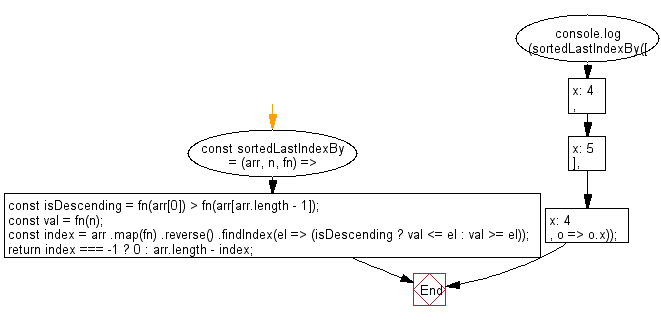# JavaScript Sorting Algorithm: Find the highest index at which a value should be inserted into an array in order to maintain its sort order, based on a provided iterator function

## JavaScript Sorting Algorithm: Exercise-17 with Solution

Write a JavaScript program to find the highest index at which a value should be inserted into an array to maintain its sort order. This is based on a provided iterator function.

Sample Solution:

JavaScript Code:

``````const sortedLastIndexBy = (arr, n, fn) => {
const isDescending = fn(arr) > fn(arr[arr.length - 1]);
const val = fn(n);
const index = arr
.map(fn)
.reverse()
.findIndex(el => (isDescending ? val <= el : val >= el));
return index === -1 ? 0 : arr.length - index;
};

console.log(sortedLastIndexBy([{ x: 4 }, { x: 5 }], { x: 4 }, o => o.x));
```
```

Sample Output:

```1
```

Flowchart:Live Demo:

See the Pen javascript-common-editor by w3resource (@w3resource) on CodePen.

Improve this sample solution and post your code through Disqus

What is the difficulty level of this exercise?

Test your Programming skills with w3resource's quiz.

﻿